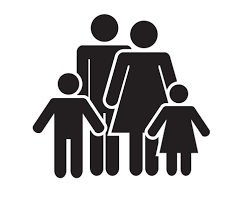# Maria 2

Maria is seven years older than her brother Robert, and in 2 years, her age will be four times Robert's age three years ago. Find their present ages.

M =  14
R =  7

### Step-by-step explanation:

M=7+R
M+2 = 4·(R-3)

M-R = 7
M-4R = -14

Row 2 - Row 1 → Row 2
M-R = 7
-3R = -21

R = -21/-3 = 7
M = 7+R = 7+7 = 14

M = 14
R = 7

Our linear equations calculator calculates it.Did you find an error or inaccuracy? Feel free to write us. Thank you!

Tips for related online calculators
Do you have a system of equations and looking for calculator system of linear equations?
Do you want to convert time units like minutes to seconds?